用户名 Email 自动登录 找回密码 密码 立即注册
 搜索 热搜: 眼底 图像 血管 视盘 分泌物

图像特效之三角几何应用

2012-6-3 17:20| 发布者: gbs| 查看: 2480| 评论: 0

 一：基本的三角函数知识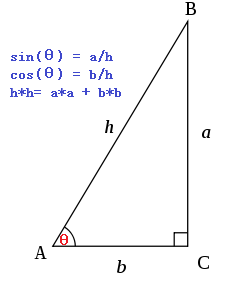同样根据a, b的值可以计算出角度θ值，称之为反三角函数，角度θ=atan2(a, b)图像处理中应用三角函数常常把中心点设置为A点，任意像素点B到A的距离可以根据三角函数来计算得出，常见的计算模型如下：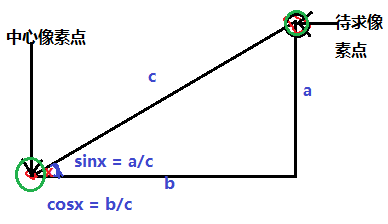对待求像素点加以一定三角函数变化，可以实现很多意想不到的图形特效，中心像素点可以通过以下计算获得int centerX = width/2;int centerY = height/2;扫描到的像素点p(x, y)可以基于 中心像素点，角度θ,两点之间距离Radius可以通过如下计算获得：int trueX = col -centerX;int trueY = row -centerY;theta = Math.atan2((trueY),(trueX));radius = Math.sqrt(trueX*trueX + trueY*trueY);二：特效原理实现的特效很简单，上述的三角几何中计算结果中，有两个可以改变其值再重新计算坐标P(x,y)。一个是角度，另外一个是半径距离，分别对角度与距离加以一定权重值计算，得到如下两种特效：1. 哈哈镜效果，主要是改变半径值，计算方法如下：double newRadius = Math.sqrt(radius) * factor;newX = centerX + (newRadius * Math.cos(theta));newY = centerY + (newRadius * Math.sin(theta));其中factor为输入参数 2. 中心螺旋效果，主要是改变角度θ的值，计算方法如下：newX = centerX + (radius * Math.cos(theta+degree * radius));newY = centerY + (radius * Math.sin(theta+degree * radius));其中degree为输入参数.三：程序效果哈哈镜效果：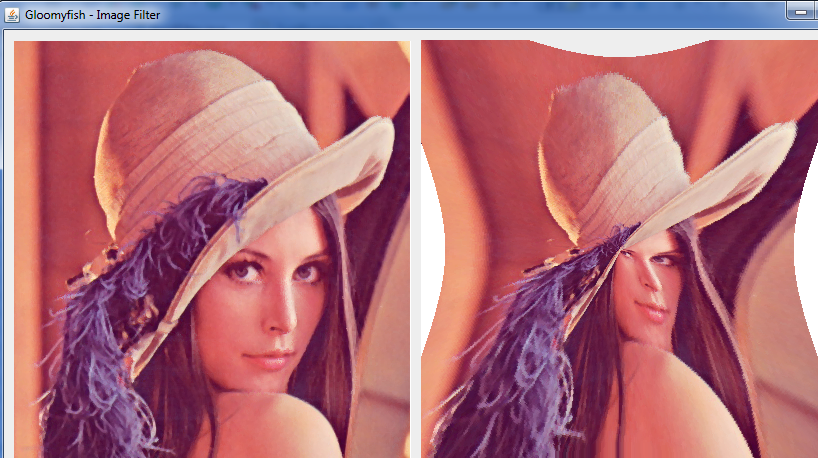螺旋效果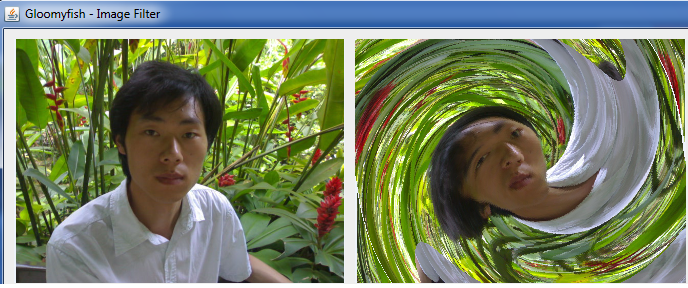相关分类|Archiver|手机版|小黑屋|爱乐眼底图像分析 ( 京ICP备1201155号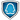GMT+8, 2019-10-19 13:03 , Processed in 0.061049 second(s), 19 queries .